### Home > AC > Chapter 14 > Lesson 14.3.1.4 > Problem3-59

3-59.
1. Use your exponent patterns to rewrite each of the expressions below. For example, if the original expression has a negative exponent, then rewrite the expression so that it has no negative exponents, and vice versa. Also, if you can simplify the expression, go ahead and do so. Note: In part (b), assume that m ≠ 0. Homework Help ✎

1. k5

2. m0

3. x−2 · x5

4.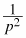5.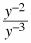6. (x2)3

7. (a2b)−1

8.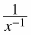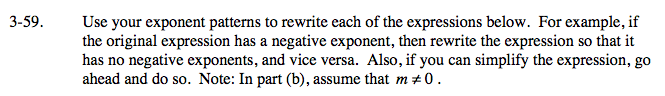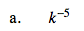$\frac{1}{k^5}$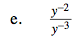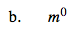1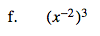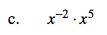x3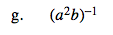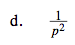p−2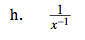Use what you know about exponents to give answers with positive exponents.